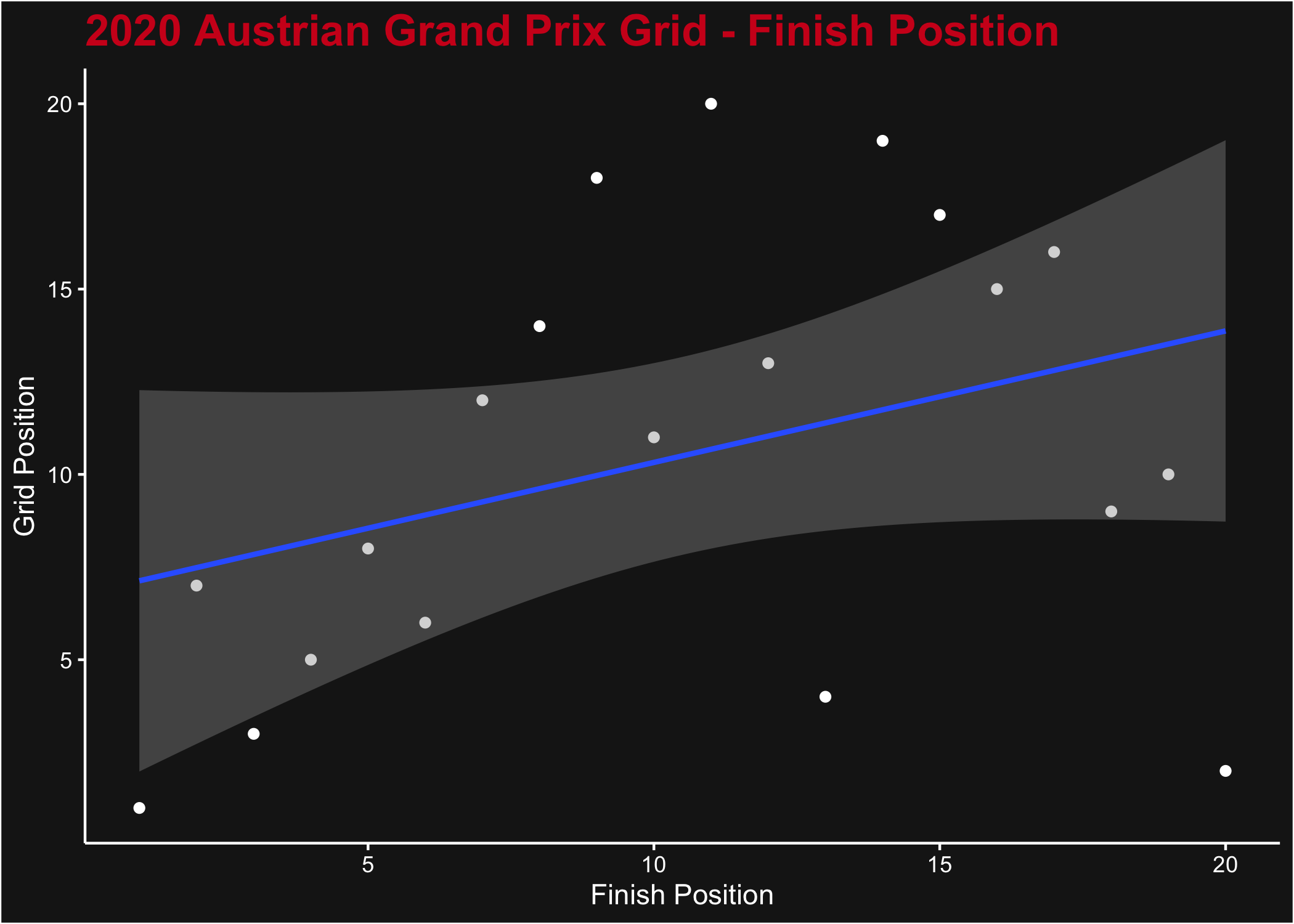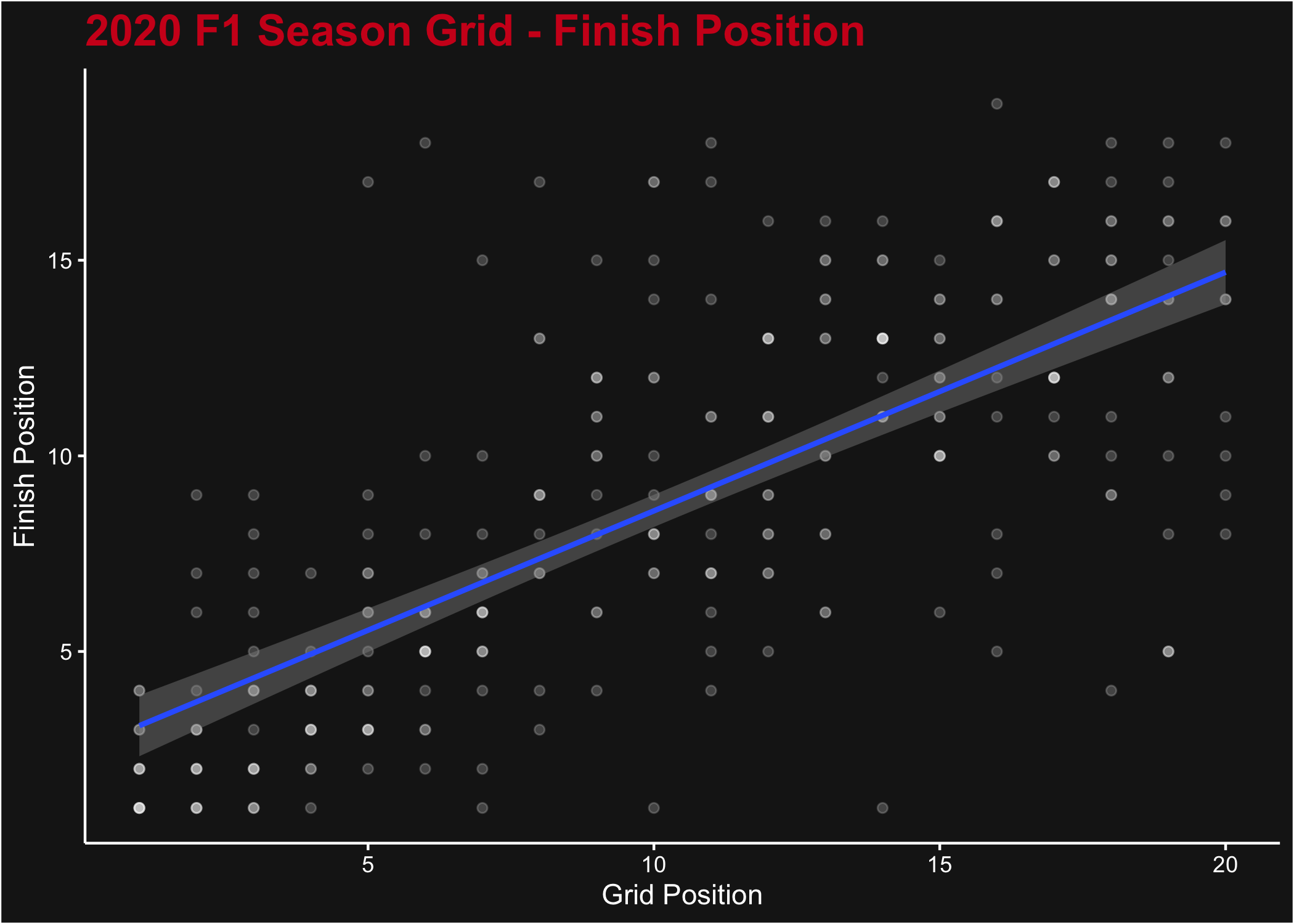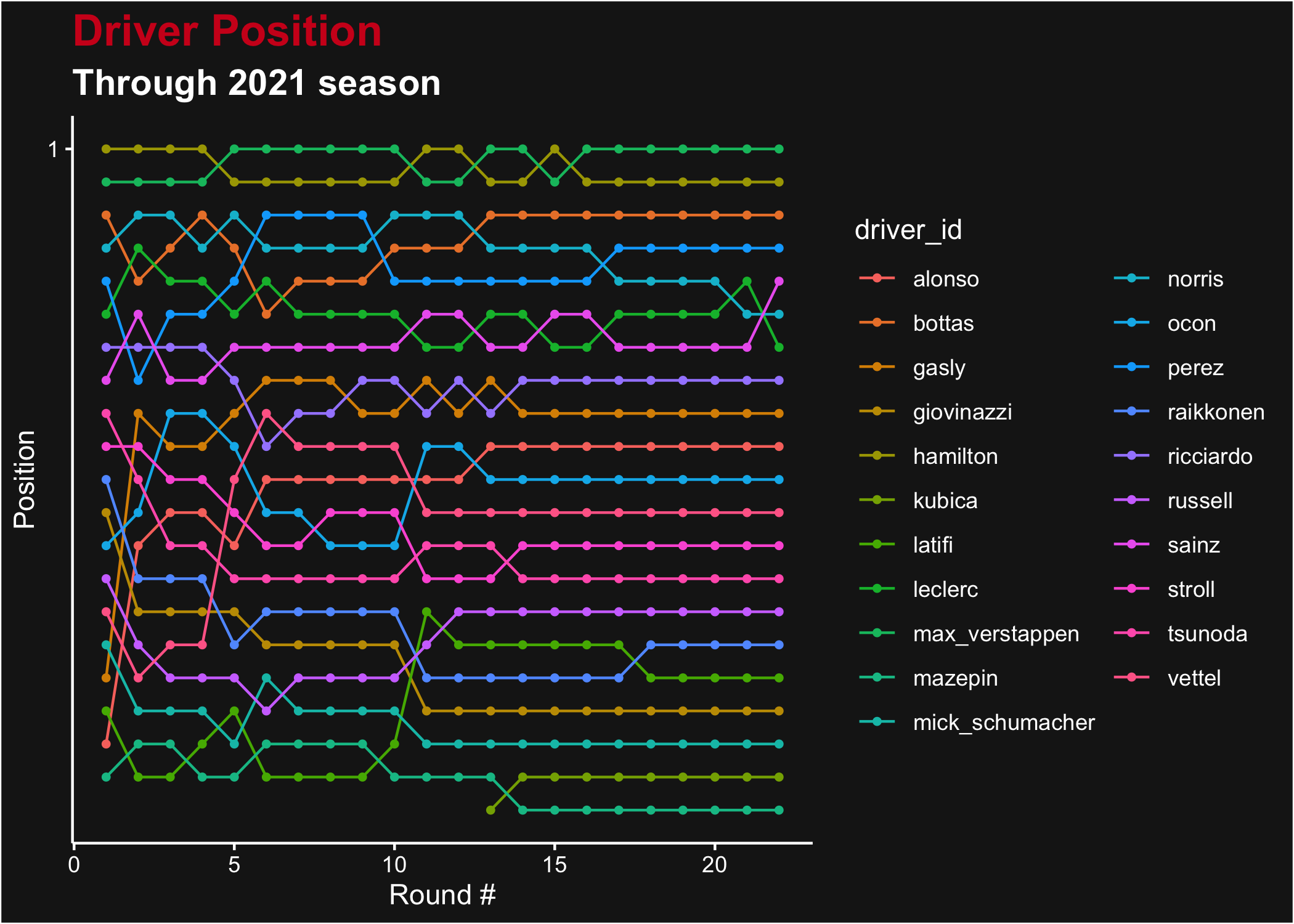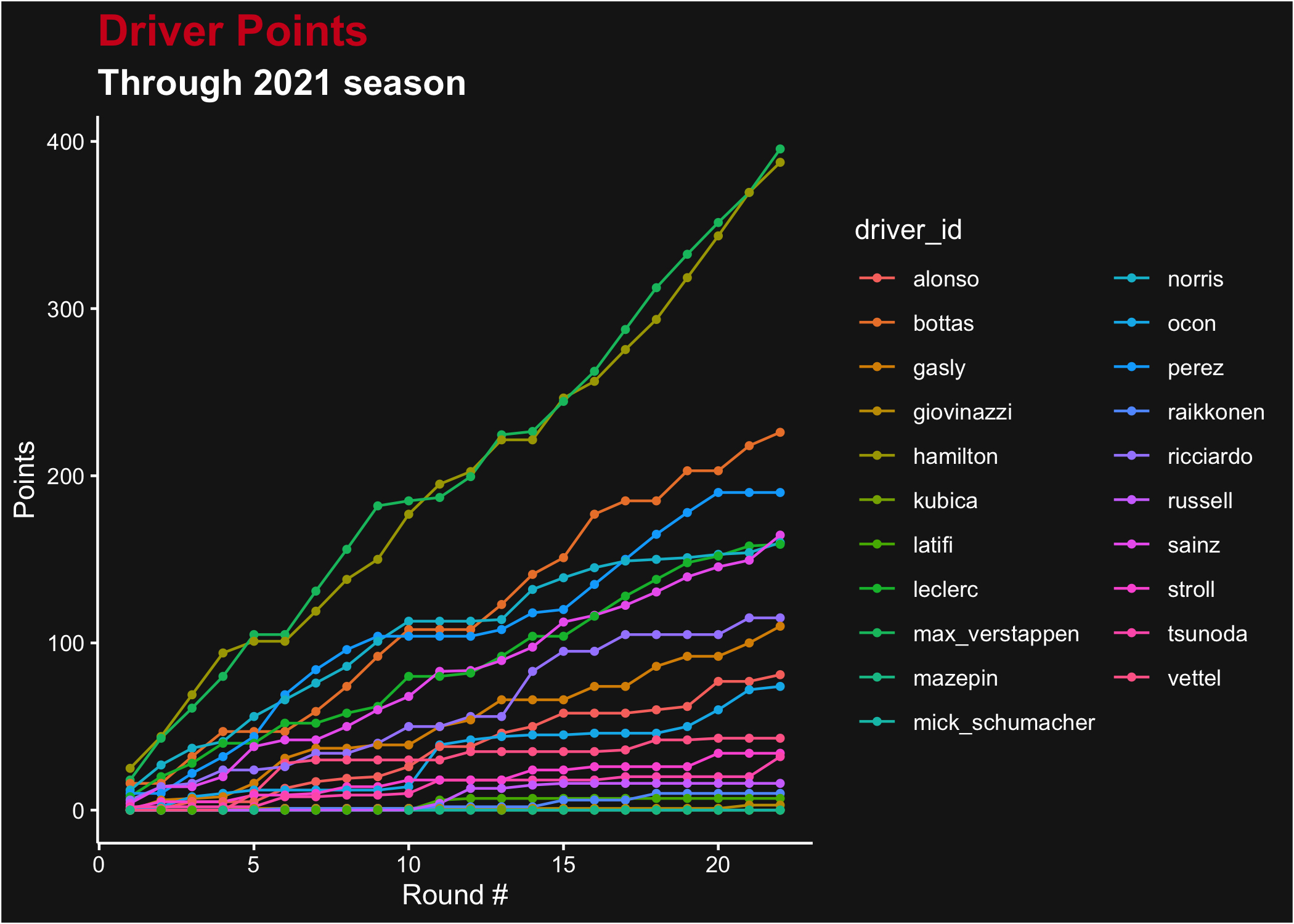# Introduction

This vignette provides a few demonstrations of possible data analysis projects using `f1dataR` and the data pulled from the Ergast API. All of the data used comes from Ergast and is not supplied by Formula 1. However, this data source is incredibly useful for a host of data.

We’ll load all the required libraries for our data analysis:

``````library(f1dataR)
library(dplyr)``````

# Sample Data Analysis

Here are a few simple data analysis examples using Ergast’s data.

Note that, when downloading multiple sets of data, we’ll put a short `Sys.sleep()` in the loop to reduce load on their servers. Please be a courteous user of their free service and have similar pauses built into your analysis code. Please read their Terms and Conditions for more informaiton.

We can make multiple repeat calls to the same function (with the same arguments) as the `f1dataR` package automatically caches responses from Ergast. You’ll see this taken advantage of in a few areas.

If you have example projects you want to share, please feel free to submit them as an issue or pull request to the `f1dataR` repository on Github.

## Grid to Finish Position Correlation

We can look at the correlation between the starting (grid) position and the race finishing position. We’ll look at the Austrian Grand Prix from 2020 for this analysis, not because of any particular reason, but that it produced a well mixed field.

``````library(ggplot2)
mutate(
grid = as.numeric(grid),
position = as.numeric(position)
)

ggplot(results, aes(x = position, y = grid)) +
geom_point(color = "white") +
stat_smooth(method = "lm") +
theme_dark_f1(axis_marks = TRUE) +
ggtitle("2020 Austrian Grand Prix Grid - Finish Position") +
xlab("Finish Position") +
ylab("Grid Position")``````plot of chunk grid_to_finish_one

Of course, this isn’t really an interesting plot for a single race. Naturally we expect that a better grid position yields a better finish position, but there’s so much variation in one race (including the effect of DNF) that it’s a very weak correlation. We can look at the whole season instead by downloading sequentially the list of results. We’ll filter the results to remove those who didn’t finish the race, and also those who didn’t start from the grid (i.e. those who started from Pit Lane, where `grid` = 0).

``````# Load the data
results <- data.frame()
for(i in seq_len(17)) {
Sys.sleep(1)
results <- dplyr::bind_rows(results, r)
}

results <- results %>%
mutate(
grid = as.numeric(grid),
position = as.numeric(position)
) %>%
filter(status %in% c("Finished", "+1 Lap", "+2 Laps", "+6 Laps"), grid > 0)

ggplot(results, aes(y = position, x = grid)) +
geom_point(color = "white", alpha = 0.2) +
stat_smooth(method = "lm") +
theme_dark_f1(axis_marks = TRUE) +
ggtitle("2020 F1 Season Grid - Finish Position") +
ylab("Finish Position") +
xlab("Grid Position")``````plot of chunk grid_to_finish_season

As expected, this produces a much stronger signal confirming our earlier hypothesis.

## Driver Points Progress

Ergast contains the points for drivers’ or constructors’ championship races as of the end of every round in a season. We can pull a season’s worth of data and compare the driver pace throughout the season, looking at both position or total points accumulation. We’ll do that for 2021, which had good competition throughout the year for P1.

``````# Load the data
points <- data.frame()
for (rnd in seq_len(22)) {
p <- load_standings(season = 2021, round = rnd) %>%
mutate(round = rnd)
points <- rbind(points, p)
Sys.sleep(1)
}

points <- points %>%
mutate(
position = as.numeric(position),
points = as.numeric(points)
)

# Plot the Results
ggplot(points, aes(x = round, y = position, color = driver_id)) +
geom_line() +
geom_point(size = 1) +
ggtitle("Driver Position", subtitle = "Through 2021 season") +
xlab("Round #") +
ylab("Position") +
scale_y_reverse(breaks = seq_along(length(unique(points\$position)))) +
theme_dark_f1(axis_marks = TRUE)``````plot of chunk round_position

What may be more interesting is the total accumulation of points. For that we can change up the plot just a little bit.

``````# Plot the Results
ggplot(points, aes(x = round, y = points, color = driver_id)) +
geom_line() +
geom_point(size = 1) +
ggtitle("Driver Points", subtitle = "Through 2021 season") +
xlab("Round #") +
ylab("Points") +
theme_dark_f1(axis_marks = TRUE)``````plot of chunk rounds_points

## Driver Laptime Scatterplot

We can look at a scatterplot of a driver’s laptimes throughout a race - possibly observing the effect of fuel usage, tire wear, pit stops, and race conditions. We’ll also show extracting constructor colour from the built-in data set.

``````# Load the laps data and select one driver (this time - Russell)
rus <- load_laps(season = 2022, round = 2) %>%
filter(driver_id == "russell")

# Get Grand Prix Name